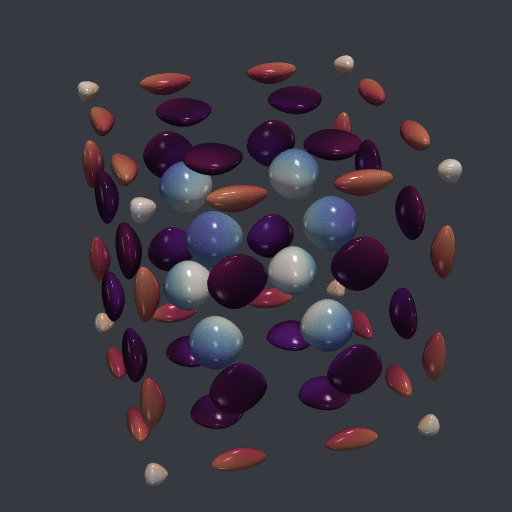Want to share your content on R-bloggers? click here if you have a blog, or here if you don't.

The gifski command-line utility is a great tool to make a GIF animation from a series of png files. At my work I’m using a laptop with Windows 10 and I don’t have admin rights. I don’t know how to install gifski on this laptop.

But gifski is also the name of a R package which wraps the gifski command-line utility, and this package can be installed without difficulty. So I used this package and the optparse package to make my own bash command gifski, which is more convenient than the package.

Here is the script:

suppressPackageStartupMessages(library("optparse"))
suppressPackageStartupMessages(library("gifski"))

option_list <- list(
make_option(
"--frames", type = "character",
help = "png files given by a glob (e.g. pic*.png)"
),
make_option(
"--fps", type = "integer", default = 20L,
help = "frames per second (default 20)"
),
make_option(
c("-l", "--loop"), type = "integer", default = 0L,
help = "number of loops, 0 for infinite (the default)",
metavar = "number"
),
make_option(
c("-s", "--size"), type = "character", default = "512x512",
help = paste0(
"size of the gif given in the form WxH where W is the width in pixels ",
"and H is the height in pixels (default 512x512)"
),
metavar = "WxH"
),
make_option(
c("-b", "--backward"), action = "store_true", default = FALSE,
help = "loop forward and backward"
),
make_option(
c("-o", "--output"), type = "character", default = "animation.gif",
help = "output gif file (default animation.gif)",
metavar = "output.gif"
)
)

opt <- parse_args(OptionParser(
option_list = option_list, prog = "gifski"
))

# check options are correct
size_ok <- grepl("^\\d.*x\\d.*$", opt$size)
if(!size_ok)
stop("Invalid 'size' option.")
if(opt$fps <= 0) stop("Invalid 'fps' option.") if(opt$loop < 0)
stop("Invalid 'loop' option.")
png_files <- Sys.glob(opt$frames) if(length(png_files) == 0L) stop("Invalid 'frames' option.") # if the user chooses the 'backward' option we duplicate the files # in a temporary directory if(opt$backward){
npngs <- 2L * length(png_files)
fmt <- paste0("pic%0", floor(log10(npngs) + 1), "d.png")
new_png_files <- file.path(tempdir(), sprintf(fmt, 1L:npngs))
file.copy(c(png_files, rev(png_files)), new_png_files)
png_files <- new_png_files
}

# get width and height
wh <- as.numeric(strsplit(opt$size, "x")[[1L]]) # a function to avoid some printed messages quiet <- function(x) { sink(tempfile()) on.exit(sink()) invisible(force(x)) } # run gifski quiet(gifski( png_files = png_files, gif_file = opt$output,
width = wh[1L],
height = wh[2L],
delay = 1/opt$fps, loop = ifelse(opt$loop == 0L, TRUE, opt$loop) )) cat("Output written to", opt$output)

Save this script where you want, say under the name gifski.R.

Now we make a bat file, say gifski.bat, which will run this script:

@echo off
echo.
C:\path\to\Rscript.exe C:\path\to\gifski.R %*

That’s all. Put this bat file in a folder available in the PATH environment variable and you can use the bash command gifski. Here is the help which is displayed by the command gifski --help:

Usage: gifski [options]

Options:
--frames=FRAMES
png files given by a glob (e.g. pic*.png)

--fps=FPS
frames per second (default 20)

-l NUMBER, --loop=NUMBER
number of loops, 0 for infinite (the default)

-s WXH, --size=WXH
size of the gif given in the form WxH where W is the width in pixels
and H is the height in pixels (default 512x512)

-b, --backward
loop forward and backward

-o OUTPUT.GIF, --output=OUTPUT.GIF
output gif file (default animation.gif)

-h, --help
Show this help message and exit

Note that there is an additional feature as compared to the original gifski tool: the --backward option, which allows to loop forward and backward.